Specific Gravity Of Solids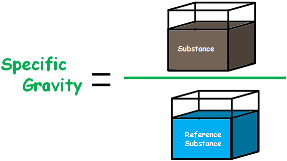Specific gravity of any substance is the ratio of the density of that substance to the density of a reference substance.

We usually take water as the reference substance.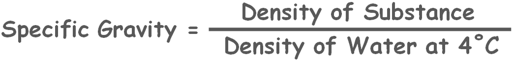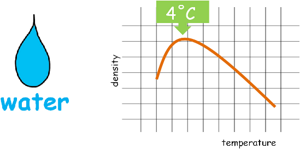But as we know density of water varies with its temperature so we take densest water which is at the temperature of four degree.

So if a substance has lower density than water, which means substance is lighter than water, then the ratio specific gravity will become less than one, simply because the numerator is smaller than denominator.

And similarly if density of that substance is higher than water, which means substance is heavier than water, then specific gravity value will become greater than one.

So by simply looking at the value of specific gravity we can estimate the weight of that substance.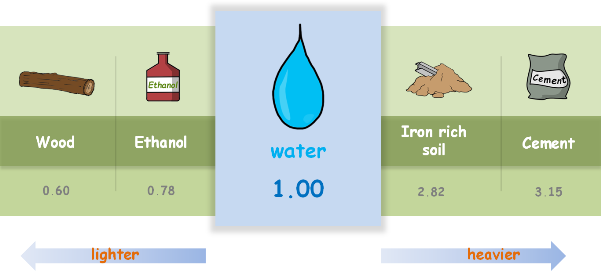If specific gravity has the value less than one than substance is lighter than water and if it is greater than one than substance is heavier than water.

The value of weight of any substance is specific gravity of that substance times the weight of water.

In case of soils that substance becomes the solids present in the soil sample.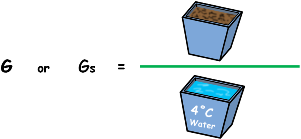So Specific gravity of solids, which is denoted by G or Gs, can be defined as the ratio of the unit weight of solids to the unit weight of water, which is weight of solids of a given volume, to the weight of equivalent volume of water at 4 degree centigrade.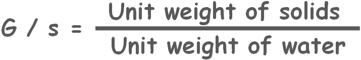For most of the soils the value of specific gravity lies between 2.65 to 2.80

In a unit volume coarse grained soils, as they have large particle size, have less mass and more voids, while in the same volume fine grained soils have more mass and less voids.

So by definition we can see that coarse grained soils will have lower value of specific gravity than fined grained soils.Similarly if organic matter is present in the soil then because of organic matter being less dense than the soil, weight of soil mass will become less and value of specific gravity will become comparatively less than the soil which doesn’t have organic matter into it.And soils with heavier elements like iron or mica will have higher weight in a given volume hence will have higher value of specific gravity.

These are the specific gravity values of different soils.Tags : specific gravity

Published on :2019-08-04